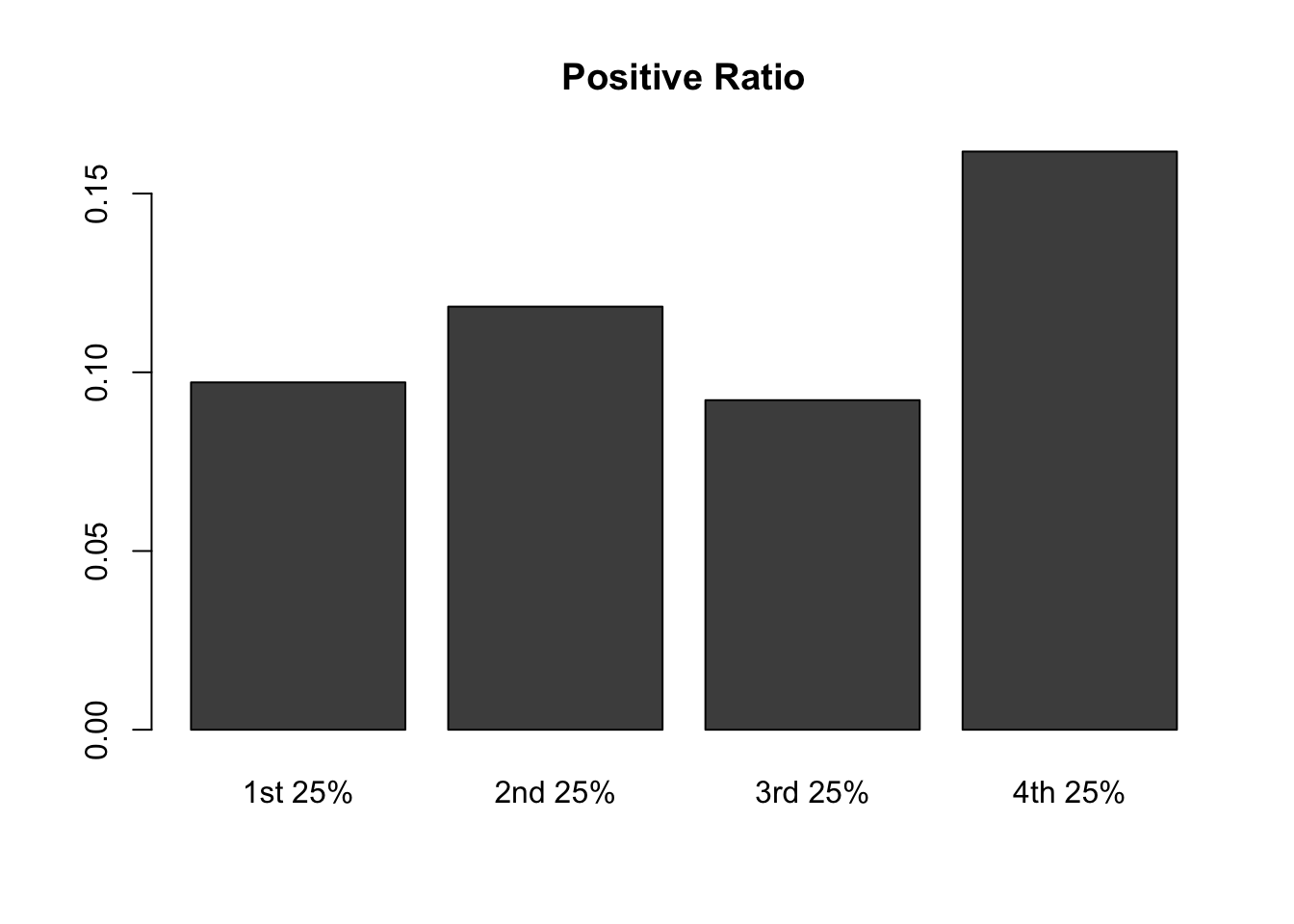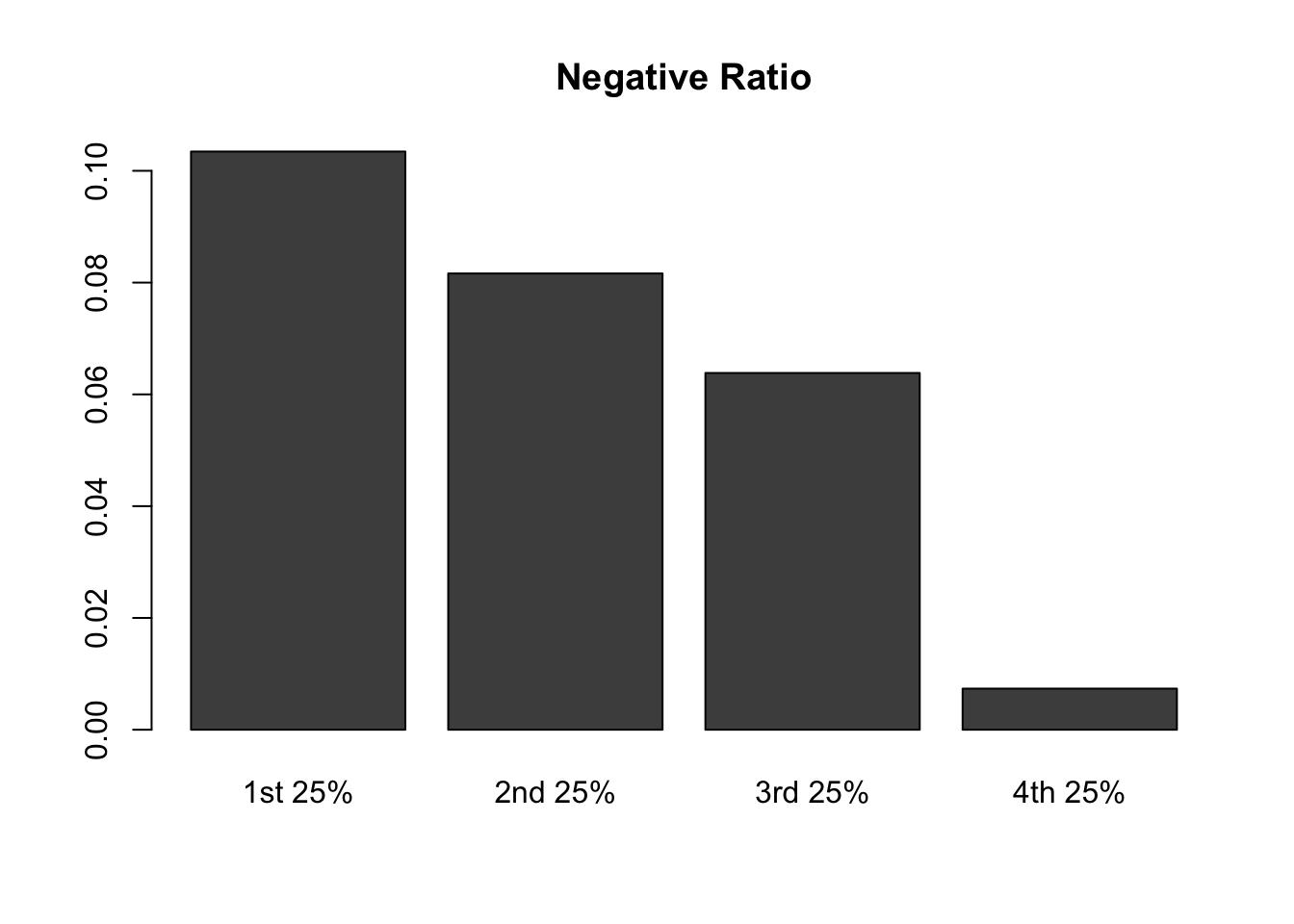#install.packages("tm")
library(tm)
## Loading required package: NLP
#install.packages("wordcloud")
library(wordcloud)
## Loading required package: RColorBrewer

# Step 1: Read in the positive and negative word files

  # 1)  Create two vectors of words
# read in positive words and assign it to a new variable called "pos"
pos <- "https://cjacks04.github.io/687/Datasets/positive-words.txt"
# scan the file and read the content into "p"
p <- scan(pos, character(0), sep = "\n")
# read in negative words and assign it to a new variable called "neg"
neg <- "https://cjacks04.github.io/687/Datasets/negative-words.txt"
# scan the file and read the content into "n"
n <- scan(neg, character(0), sep = "\n")

# 2) Clean Data
# remove useless rows (row 1 to row 34) of "p"
#p <- p[-c(1:34)]
# remove useless rows (row 1 to row 34) of "n"
#n <- n[-c(1:34)]
# check the cleaned data sets "p" and "n"
head(p,50)
##   "a+"              "abound"          "abounds"
##   "abundance"       "abundant"        "accessable"
##   "accessible"      "acclaim"         "acclaimed"
##  "accommodative"   "accomodative"    "accomplish"
##  "accomplished"    "accomplishment"  "accomplishments"
##  "accurate"        "accurately"      "achievable"
##  "achievement"     "achievements"    "achievible"
##  "advantage"       "advantageous"
  head(n,50)
##   "2-faced"       "2-faces"       "abnormal"      "abolish"
##   "abominable"    "abominably"    "abominate"     "abomination"
##   "abort"         "aborted"       "aborts"        "abrade"
##  "abrasive"      "abrupt"        "abruptly"      "abscond"
##  "absence"       "absent-minded" "absentee"      "absurd"
##  "absurdity"     "absurdly"      "absurdness"    "abuse"
##  "abused"        "abuses"        "abusive"       "abysmal"
##  "abysmally"     "abyss"         "accidental"    "accost"
##  "accursed"      "accusation"    "accusations"   "accuse"
##  "accuses"       "accusing"      "accusingly"    "acerbate"
##  "acerbic"       "acerbically"   "ache"          "ached"
##  "aches"         "achey"         "aching"        "acrid"
##  "acridly"       "acridness"

# Step 2: Process in the MLK speech

# 3)    Read the text file
# read in text file "MLK"
# remove all blank lines in the text
mlk <- mlk[which(mlk != "")]

# 4)  Create a term matrix
# interprets each element of the "mlk" as a document and create a vector source
words.vec <- VectorSource(mlk)
# create a Corpus, a "Bag of Words"
words.corpus <- Corpus(words.vec)
# first step transformation: make all of the letters in "words.corpus" lowercase
words.corpus <- tm_map(words.corpus, content_transformer(tolower))
## Warning in tm_map.SimpleCorpus(words.corpus, content_transformer(tolower)):
## transformation drops documents
  # second step transformation: remove the punctuation in "words.corpus"
words.corpus <- tm_map(words.corpus, removePunctuation)
## Warning in tm_map.SimpleCorpus(words.corpus, removePunctuation):
## transformation drops documents
  # third step transformation: remove numbers in "words.corpus"
words.corpus <- tm_map(words.corpus, removeNumbers)
## Warning in tm_map.SimpleCorpus(words.corpus, removeNumbers): transformation
## drops documents
  # final step transformation: take out the "stop" words, such as "the", "a" and "at"
words.corpus <- tm_map(words.corpus, removeWords, stopwords("english"))
## Warning in tm_map.SimpleCorpus(words.corpus, removeWords,
## stopwords("english")): transformation drops documents
  # create a term-document matrix "tdm"
tdm <- TermDocumentMatrix(words.corpus)
# view term-document matrix "tdm"
tdm
## <<TermDocumentMatrix (terms: 463, documents: 29)>>
## Non-/sparse entries: 682/12745
## Sparsity           : 95%
## Maximal term length: 14
## Weighting          : term frequency (tf)
  # 5)  Create a list of counts for each word
# convert tdm into a matrix called "m"
m <- as.matrix(tdm)
# create a list of counts for each word named "wordCounts"
wordCounts <- rowSums(m)
# sort words in "wordCounts" by frequency
wordCounts <- sort(wordCounts, decreasing=TRUE)
# check the first several items in "wordCounts" to see if it is built correctly
head(wordCounts)
##    will freedom   negro     one     let    ring
##      26      20      13      13      13      12

# Step 3: Determine how many positive words were in the speech

# 6)    Scale the number based on the total number of words in the speech
# sum the total number of words and store the value to "totalWords"
totalWords <- sum(wordCounts)
# create a vector "words" that contains all the words in "wordCounts"
words <- names(wordCounts)
# locate which words in "mlk" were positive (appeared in positive-word list)
matchedP <- match(words, p, nomatch = 0)
# calculate the total number of positive words in "mlk" speech and assign the number to the variable "pTotal"
pTotal <- sum(wordCounts[which(matchedP != 0)])
# view the total number of positive words (95 positive words in the speech)
pTotal 
##  95
  # view the percentage of positive words (11.29608% of the speech words are positive)
pTotal/totalWords 
##  0.1129608

# Step 4: Determine how many negative words were in the speech

 # 8)   Scale the number based on the total number of words in the speech
# locate which words in "mlk" were negative (appeared in negative-word list)
matchedN <- match(words, n, nomatch = 0)
# calculate the total number of negative words in "mlk" speech and assign the number to a variable named "nTotal"
nTotal <- sum(wordCounts[which(matchedN != 0)])
# view the total number of negative words (63 negative words)
nTotal
##  63
  # view the percentage of negative words (7.4911% of the speech words are negative)
nTotal/totalWords
##  0.07491082

# Step 5: Redo the positive and negative calculations for each 25% of the speech

# define a cutpoint to split the document into 4 parts; round the number to get an interger
cutpoint <- round(length(words.corpus)/4)

# first 25%
# create word corpus for the first quarter using cutpoints
words.corpus1 <- words.corpus[1:cutpoint]
# create term document matrix for the first quarter
tdm1 <- TermDocumentMatrix(words.corpus1)
# convert tdm1 into a matrix called "m1"
m1 <- as.matrix(tdm1)
# create a list of word counts for the first quarter and sort the list
wordCounts1 <- rowSums(m1)
wordCounts1 <- sort(wordCounts1, decreasing=TRUE)
# calculate total words of the first 25%
totalWords1 <- sum(wordCounts1)
# create a vector that contains all the words in "wordCounts1"
words1 <- names(wordCounts1)
# locate which words in first quarter were positive (appeared in positive-word list)
matchedP1 <- match(words1, p, nomatch = 0)
# calculate the number of positive words in first quarter
ptotalNumber1 <- sum(wordCounts1[which(matchedP1 != 0)])
# calculate the ratio of positive words in first quarter
ratiop1 <- ptotalNumber1/totalWords1
# locate which words in first quarter were negative (appeared in negative-word list)
matchedN1 <- match(words1, n, nomatch = 0)
# calculate the number of negative words in first quarter
ntotalNumber1 <- sum(wordCounts1[which(matchedN1 != 0)])
# calculate the ratio of negative words in first quarter
ration1 <- ntotalNumber1/totalWords1

# second 25%
# create word corpus for the second quarter using cutpoints
words.corpus2 <- words.corpus[(cutpoint+1):(2*cutpoint)]
# create term document matrix for the second quarter
tdm2 <- TermDocumentMatrix(words.corpus2)
m2 <- as.matrix(tdm2)
# create a list of word counts for the second quarter and sort the list
wordCounts2 <- rowSums(m2)
wordCounts2<- sort(wordCounts2, decreasing=TRUE)
# calculate total words of the second 25%
totalWords2 <- sum(wordCounts2)
# create a vector that contains all the words in "wordCounts2"
words2 <- names(wordCounts2)
# locate which words in second quarter were positive (appeared in positive-word list)
matchedP2 <- match(words2, p, nomatch = 0)
# calculate the number of positive words in second quarter
ptotalNumber2 <- sum(wordCounts2[which(matchedP2 != 0)])
# calculate the ratio of positive words in second quarter
ratiop2 <- ptotalNumber2/totalWords2
# locate which words in second quarter were negative (appeared in negative-word list)
matchedN2 <- match(words2, n, nomatch = 0)
# calculate the number of negative words in second quarter
ntotalNumber2 <- sum(wordCounts2[which(matchedN2 != 0)])
# calculate the ratio of negative words in second quarter
ration2 <- ntotalNumber2/totalWords2

# third 25%
# create word corpus for the third quarter using cutpoints
words.corpus3 <- words.corpus[(2*cutpoint+1):(3*cutpoint)]
# create term document matrix for the third quarter
tdm3 <- TermDocumentMatrix(words.corpus3)
m3 <- as.matrix(tdm3)
# create a list of word counts for the third quarter and sort the list
wordCounts3 <- rowSums(m3)
wordCounts3<- sort(wordCounts3, decreasing=TRUE)
# calculate total words of the third 25%
totalWords3 <- sum(wordCounts3)
# create a vector that contains all the words in "wordCounts3"
words3 <- names(wordCounts3)
# locate which words in third quarter were positive (appeared in positive-word list)
matchedP3 <- match(words3, p, nomatch = 0)
# calculate the number of positive words in third quarter
ptotalNumber3 <- sum(wordCounts3[which(matchedP3 != 0)])
# calculate the ratio of positive words in third quarter
ratiop3 <- ptotalNumber3/totalWords3
# locate which words in third quarter were negative (appeared in negative-word list)
matchedN3 <- match(words3, n, nomatch = 0)
# calculate the number of negative words in third quarter
ntotalNumber3 <- sum(wordCounts3[which(matchedN3 != 0)])
# calculate the ratio of negative words in third quarter
ration3 <- ntotalNumber3/totalWords3

# forth 25%
# create word corpus for the forth quarter using cutpoints
words.corpus4 <- words.corpus[(3*cutpoint+1):length(words.corpus)]
# create term document matrix for the forth quarter
tdm4 <- TermDocumentMatrix(words.corpus4)
m4 <- as.matrix(tdm4)
# create a list of word counts for the forth quarter and sort the list
wordCounts4 <- rowSums(m4)
wordCounts4<- sort(wordCounts4, decreasing=TRUE)
# calculate total words of the forth 25%
totalWords4 <- sum(wordCounts4)
# create a vector that contains all the words in "wordCounts4"
words4 <- names(wordCounts4)
# locate which words in forth quarter were positive (appeared in positive-word list)
matchedP4 <- match(words4, p, nomatch = 0)
# calculate the number of positive words in forth quarter
ptotalNumber4 <- sum(wordCounts4[which(matchedP4 != 0)])
# calculate the ratio of positive words in forth quarter
ratiop4 <- ptotalNumber4/totalWords4
# locate which words in forth quarter were negative (appeared in negative-word list)
matchedN4 <- match(words4, n, nomatch = 0)
# calculate the number of negative words in forth quarter
ntotalNumber4 <- sum(wordCounts4[which(matchedN4 != 0)])
# calculate the ratio of negative words in forth quarter
ration4 <- ntotalNumber4/totalWords4

# 10) Compare the results
# combine positive words ratio of four quarters into one dataframe
ratioP <- cbind(ratiop1, ratiop2, ratiop3, ratiop4)
# combine negative words ratio of four quarters into one dataframe
ratioN <- cbind(ration1, ration2, ration3, ration4)
# create a bar plot for the positive ratios
barplot(ratioP, names.arg = c("1st 25%","2nd 25%","3rd 25%","4th 25%"), main = "Positive Ratio")# create a bar plot for the negative ratios
barplot(ratioN, names.arg = c("1st 25%","2nd 25%","3rd 25%","4th 25%"), main = "Negative Ratio")